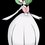# Solving Pell Equation of Norms other than -1 and 1

People, I crossed a Pell-type equation: x^2 - 6y^2 = 3 which it has norm 3. Are there ways to solve this equation without using concepts from Abstract Algebra such as factoring in a number field or what?Note by John Ashley Capellan
6 years, 10 months ago

This discussion board is a place to discuss our Daily Challenges and the math and science related to those challenges. Explanations are more than just a solution — they should explain the steps and thinking strategies that you used to obtain the solution. Comments should further the discussion of math and science.

When posting on Brilliant:

• Use the emojis to react to an explanation, whether you're congratulating a job well done , or just really confused .
• Ask specific questions about the challenge or the steps in somebody's explanation. Well-posed questions can add a lot to the discussion, but posting "I don't understand!" doesn't help anyone.
• Try to contribute something new to the discussion, whether it is an extension, generalization or other idea related to the challenge.
• Stay on topic — we're all here to learn more about math and science, not to hear about your favorite get-rich-quick scheme or current world events.

MarkdownAppears as
*italics* or _italics_ italics
**bold** or __bold__ bold
- bulleted- list
• bulleted
• list
1. numbered2. list
1. numbered
2. list
Note: you must add a full line of space before and after lists for them to show up correctly
paragraph 1paragraph 2

paragraph 1

paragraph 2

[example link](https://brilliant.org)example link
> This is a quote
This is a quote
    # I indented these lines
# 4 spaces, and now they show
# up as a code block.

print "hello world"
# I indented these lines
# 4 spaces, and now they show
# up as a code block.

print "hello world"
MathAppears as
Remember to wrap math in $$ ... $$ or $ ... $ to ensure proper formatting.
2 \times 3 $2 \times 3$
2^{34} $2^{34}$
a_{i-1} $a_{i-1}$
\frac{2}{3} $\frac{2}{3}$
\sqrt{2} $\sqrt{2}$
\sum_{i=1}^3 $\sum_{i=1}^3$
\sin \theta $\sin \theta$
\boxed{123} $\boxed{123}$

## Comments

Sort by:

Top Newest

You can read up on Pell's Equation, to understand how to generate solutions from a base case.

1) Observe that $(5,2)$ is the first non-trivial solution to $x^2 - 6y^2 = 1$.

2) Observe that

$( a^2 - 6b^2 ) ( c^2 - 6 d^2 ) = a^2c^2 + 36 b^2d^2 - 6 b^2 c^2 - 6a^2d^2= ( ac + 6bd) ^2 - 6 ( bc+ad) ^2.$

As such, we define pair-multiplication as $(a,b) \otimes (c, d) = ( ac + 6 bd , bc + ad)$.

3) Observe that $(3, 1)$ is a solution to $x^2 - 6y^2 = 3$.

4) Hence, solutions exist in the form of $(3,1) \otimes ( 5,2)^ n$, where $n$ is a non-negative integer.
For example, with $n=1$, we get $(3,1) \otimes (5,2) = ( 15 + 12 , 5 + 6) = (27, 11)$. We can check that $27^2 - 6 \times 11^2 = 729 - 726 = 3$.

Followup question: Are there other solutions? (ignore negative values)

Staff - 6 years, 10 months ago

Log in to reply

It's $(ac+6bd,bc+ad)$. Fix it to avoid confusion.

- 6 years, 5 months ago

Log in to reply

You also left out an $a^2$ in Point 2. Cheers, G.

- 2 years, 10 months ago

Log in to reply

Fixed. Thanks!

Staff - 2 years, 10 months ago

Log in to reply

@John Ashley Capellan Can you add what you learnt about Pell's Equation to the Wiki? Thanks!

Staff - 6 years, 8 months ago

Log in to reply

First find the smallest positive solution of x,y. Express it as x+6^0.5y Then find solution of the equation x^2-6y^2=1. Express it as x+6^0.5y Multiply any of these two you get another solution. Hence obtain all solutions.

- 6 years, 10 months ago

Log in to reply

Could you please explain in a bit more detail? Sorry for the trouble.

- 6 years, 10 months ago

Log in to reply

You can know about my solution by searching pell fermat equation in wikipedia

- 6 years, 10 months ago

Log in to reply

×

Problem Loading...

Note Loading...

Set Loading...